Precalculus : Solve a Triangle in Which 3 Sides Are Given (SSS)

Example Questions

Example Question #1 : Solve A Triangle In Which 3 Sides Are Given (Sss)

Which is NOT an angle of the following triangle?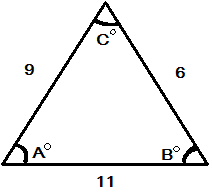(Not drawn to scale.)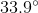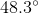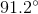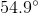Explanation:

In order to solve this problem, we need to find the angles of the triangle. Only then will we be able to find which answer choice is NOT an angle. Using the Law of Cosines we are able to find each angle.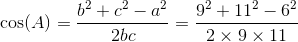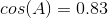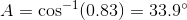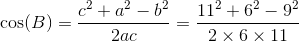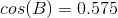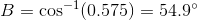To find anglewe use the formula again or we can remember that the angles in a triangle add up to.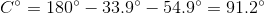The answer choice that isn't an actual angle of the triangle is.

Example Question #1 : Solve A Triangle In Which 3 Sides Are Given (Sss)

Solve the triangle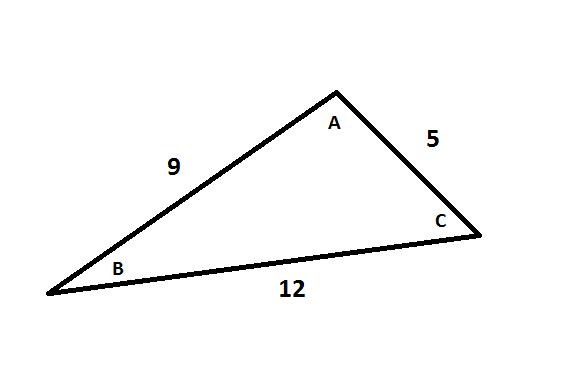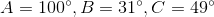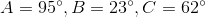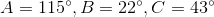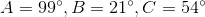Explanation:

Since we are given all 3 sides, we can use the Law of Cosines in the angle form: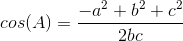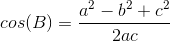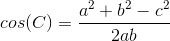Let's start by finding angle A: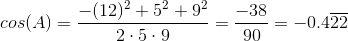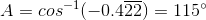Now let's solve for B: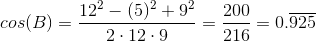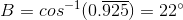We can solve for C the same way, but since we now have A and B, we can use our knowledge that all interior angles of a triangle must add up to 180 to find C.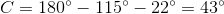Example Question #3 : Solve A Triangle In Which 3 Sides Are Given (Sss)

Given three sides of the triangle below, determine the angles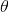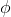, and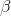in degrees.Explanation:

We are only given sides, so we must use the Law of Cosines. The equation for the Law of Cosines is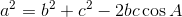,

whereandare the sides of a triangle and the angleis opposite the side.

We have three known sides and three unknown angles, so we must write the Law three times, where each equation lets us solve for a different angle.

To solve for angle, we write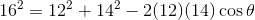and solve forusing the inverse cosine function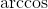on a calculator to get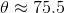.

Similarly, for angle,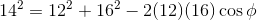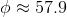and for,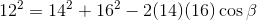and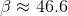Example Question #1 : Solve A Triangle In Which 3 Sides Are Given (Sss)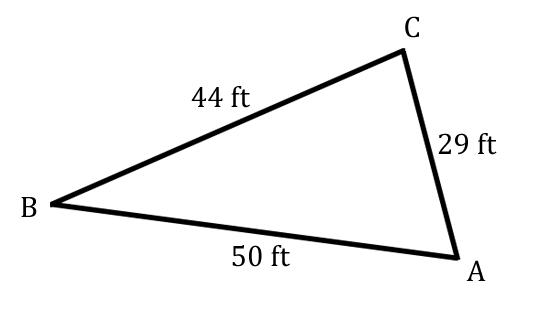What is the measurement of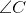? Round to the nearest tenth, if needed.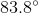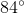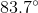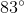Explanation:

We need to use the Law of Cosines for sidethen solve for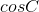.

Therefore,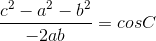.

Plugging in the information provided, we have: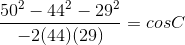.

Then simplify,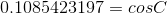.

To solve for, use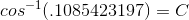.

Solve and then round to the appropriate units: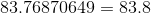. Therefore,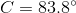.

Example Question #1 : Solve A Triangle In Which 3 Sides Are Given (Sss)

Find the measure, in degrees, of the largest angle in a triangle whose sides measure,, and.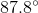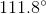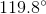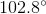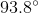Explanation:

When all three sides are given, Law of Cosines is appropriate.

Since 10 is the largest side length, it is opposite the largest angle and thus should be the c-value in the equation below.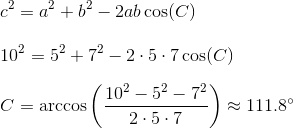All Precalculus Resources